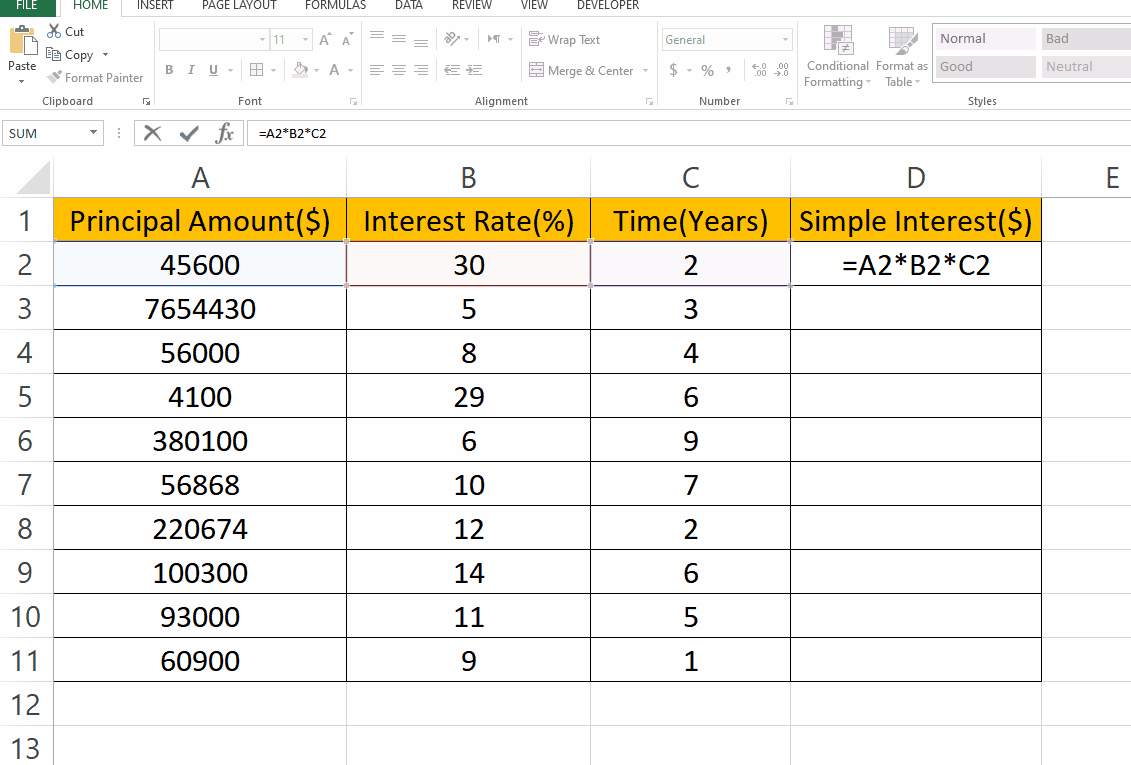# How to calculate Simple Interest in ExcelWhen you deposit money in a savings account or borrow money from a lender, you are usually subject to interest charges. Interest is a fee paid for the use of someone else’s money, and it can be calculated in different ways depending on the terms of the loan or investment. In this tutorial we will learn how to calculate Simple interest in Excel. To find Simple Interest the dataset we will be working on contains 3 columns which are Principal Amount (\$), Interest Rate (%) and Time (Years). Follow the steps below for finding simple interest.

### Step 1 – Formula for Simple Interest:– To find Simple Interest type the formula, syntax is
=P*R*T
P is for Principal Amount
R is for Interest Rate
T is for Time Period
– In our case the formula is
=A2*B2*C2

### Step 2 – Find Simple Interest for the rest of the values:– To find simple interest for the rest of the values accordingly drag the cell with formula from bottom right to the last cell.